Hex Maze

Published on
19,305 Points
8,405 Views
14 Endorsements
Awarded
This article describes a JavaScript program that creates a maze made of hexagonal cells.  In Part 2, we'll extend the program by adding a depth-first search algorithm that solves the maze.  The Hex Maze program is unusual and visually impressive since you can watch the action as the maze is created and solved.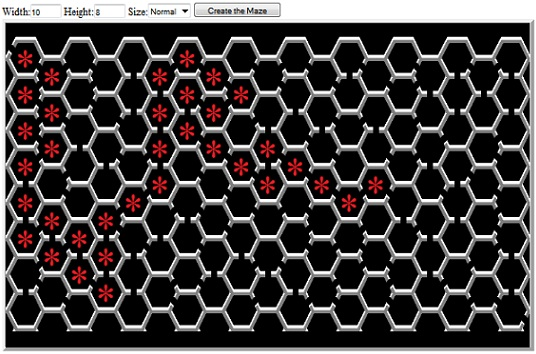Hexagons connect up into a regular lattice in nature and they are of scientific interest for many reasons.  Bees use hexagonal honeycombs because they enclose the maximum volume of honey with the minimum amount of beeswax and construction labor.  Carbon atoms naturally attach themselves in hexagonal patterns.  Throw in a few pentagons and you have buckmisterfullerine, one of my favorite molecules.  Have you ever noticed that the stars spangling "Ol' Glory" are arranged in a hexagonal pattern?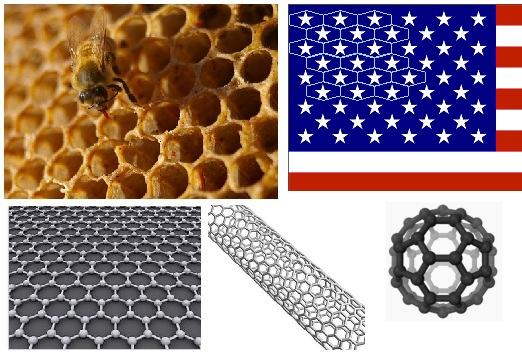Note:  There is an iconic Experts-Exchange question that relates to hexagonal cells.  It is historically significant because it involved a large number of top EE Experts (the aptly-named "A Team") working together while at Core Conference, 2008. See my LYIR 2008 posting for more on that, including a link to the original question and photos.
I first got interested in hexagonal cells back in 1979.  I had written a BASIC program to create a maze and it occurred to me that a maze built from hexagonal cells would be an interesting challenge. I wrote and published an article about it in a magazine named Creative Computing  I recently stumbled upon a copy of that old program (see my EE Blog entry) and, as a bit of recreational programming, I decided to revisit the challenge in JavaScript.

Mapping the Cells

The hard part about working with hexagonal cells is that although the pattern is perfectly regular, the cells just don't match up to elements of a regular (rectangular) 2-dimensional array.  This is a problem, not just with displaying the entire array, but in keeping track of a "current position" and moving from cell to cell.

In a square-celled maze, each array element is intuitively positioned directly next to its adjacent cells.  For instance, (x+1,y) is the cell to the right and (x,y+1) is the cell directly below.

But the cells in a hex maze do not align with a simple matrix.  Each cell has six neighbors and the rows are differing lengths (odd-numbered rows have one fewer cells).  The program needs to be able to move from cell-to-cell in any of six directions and be able to query each neighbor before moving.  It also needs to take care to avoid boundary faults (moving beyond the edge).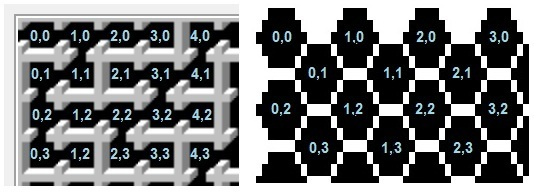In the original 1980 "Bee Amazed" BASIC program, I used a layout like that on the right side of the figure, which required a bunch of odd-line vs. even-line logic.  I was never really happy with that (even back then), so this time, I tried some alternatives.  One interesting option is to work with a 3-dimensional array, where the odd (shorter) lines are tracked as if they are behind the even lines.  But that layout ended up with its own set of what I felt were kludgey (inelegant) complications.

I eventually worked the problem in reverse: The simplest, most intuitive cell-to-cell movement logic would be if I could adjust like this:

Northeast:    X +1,   Y -1
Southeast:   X +1,   Y +1
Southwest:   X -1,   Y +1
Northwest:    X -1,   Y -1

...regardless of whether the starting cell was on an odd or even row.  So I penciled a honeycomb on some graph paper, and labeled the cells with the desired array coordinates.  What emerged was a mapping that I'd never have thought of while coding for a 16KB TRS-80 because it wastes a lot of array space: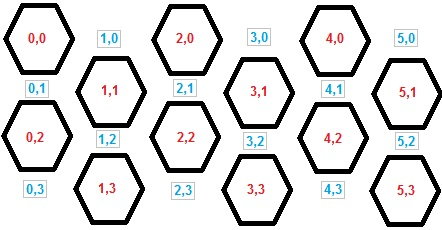The trick is to ignore half of the 2-D matrix, scattering unused elements at regular intervals.  That way, the "motion delta" logic for moving NE, SE, SW, SE is consistent for every cell.  And the only "complication" is that to move directly N or S, I'd need to adjust Y by 2:

South:    X +0,  Y +2
North:    X +0,  Y -2

This sparse array approach was 31 years in the making, but the result is simple and elegant and it illustrates the value of taking a fresh look at an old problem.

Maze Representation in Memory

Each hexagonal cell is represented by one element of the array, and bit flags are used to indicate where there are doors.

With a square maze, you can use Up, Down, Left, and Right, but for the hex maze, I used compass points, cardinal and intercardinal directions: N, NE, SE, S, SW, and NW.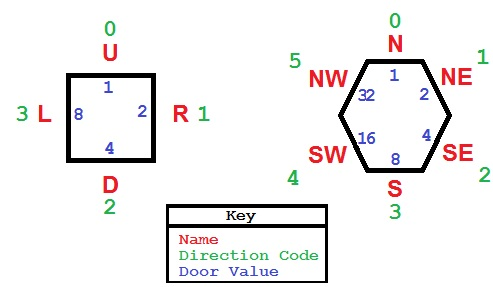I assigned a set of constants for symbolic reference (DIR_xx is a DIRection of motion, and the DOOR_xx values are corresponding flags to indicate when there is a door in that direction (otherwise there is a wall):
//------------------------------ constants
DIR_N=0;   DIR_NE=1;  DIR_SE=2;  DIR_S=3;  DIR_SW=4;   DIR_NW=5;

DOOR_N=1;  DOOR_NE=2; DOOR_SE=4; DOOR_S=8; DOOR_SW=16; DOOR_NW=32;
For instance, if the cell value is 1, there is a door to the North.  If the cell value is 8, there is a door to the South.  It the cell value is 9, then there are doors in both the N and S sides.  This technique lets me represent any combination of walls and doors with a single integer value.  If you want to learn more about using bit flags in your programming, see
Binary Bit Flags: Tutorial and Usage Tips.

Displaying the Maze

JavaScript contains no direct graphical functions, and the way I have rendered a square maze in the past is to manipulate the IMG elements in the HTML DOM.  With a square maze, that's pretty easy, but these hexagonal cells took a bit more work.  I ended up breaking each cell into component pieces.

I used MsPaint to draw a maze, adding highlights to give it a 3-D effect and tweaking the pixels until it looked the way I wanted it to look when there were doors in each of the walls.  I then sliced up my reference maze and saved the unique pieces as separate image files.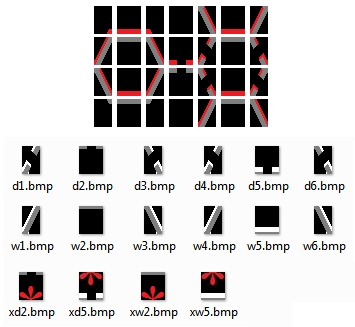In the program, I build up a long string of HTML that looks like this:
<img HEIGHT=28 src='images/w1.bmp"><img HEIGHT=28 src='images/w2.bmp"><img HEIGHT=28 src='images/w2.bmp"> ... <br>
<img HEIGHT=28 src='images/w3.bmp"><img HEIGHT=28 src='images/w5.bmp"><img HEIGHT=28 src='images/w4.bmp"> ... <br>
...etc...
When done, I insert the entire string as the innerHTML of a <TD> element.  It would also be possible to create the IMG elements directly, but I find that generating HTML strings is easier to code and easier to debug.

Each row of images is actually half of a row of cells, so in creating the image HTML, I draw the top half then the bottom half in the same loop iteration.  That also draws the top half of the odd-numbered lines.  I don't need to handle the odd-numbered lines separately because when there is a door on top of a cell, then there is also a door on the bottom of the cell above it (and a NW door is handled by the SE door from the cell above, etc.)

A maze initialized to all 0s is a simple honeycomb (or sheet of pure carbon graphene, if you prefer) with all walls.  I wanted to be able to watch as the maze is created (and solved), so I also wrote a function that updates the on-screen images when the cell values change.  I call that SetCellImg() function to update the IMAGES array in the DOM to reflect the current state of any cell, given its X,Y coordinates.

Maze Generation Algorithm

This is virtually identical to the code in my 1980 BASIC version.  It uses the same logic as for a square maze, with the one significant difference that there are six, rather than four, possible exits from a cell.  Here is the algorithm, described simply:

1) Start in a random location (X,Y).
2) Check the neighboring cells:  Make a list of neighbors that have never been visited (i.e., have no doors).  When done, the list contain up to six possible directions to move.
3) If the list is empty (you are stuck), scan to locate any cell that has been visited that is next to a cell that has not.  Set that as the current cell and go to 2.
4) Choose randomly from that list of available directions.
5) Set the corresponding door for the current cell.
6) Move into the new cell (update X,Y) and set the corresponding door for the new cell.
7) If all cells have at least one door, we're done.  Otherwise go to 2.

The algorithm ensures that there is a single path between any two cells.  It's easy to verify that when you watch as the maze is updated in realtime:  A "corridor" winds around randomly, growing longer and longer until it curls itself into a dead end.  At that point, one of the cells along that route sprouts a new offshoot and a new corridor begins to grow.  The "scanning logic" (step 3) insures that each new branch is connected to the original branch at exactly one point.  There are no "islands" of disconnected cells and no "extra" connections that could lead to multiple paths through the maze.

There are other ways to create mazes (see the Wikipedia entry), but this one yields good result and is easy to implement.

JavaScript Programming Notes

The original code uses nothing but global variables (of course:  It's in circia 1979 BASIC!) but with this version, I was able to use an object-oriented approach.  Most of the maze-handling is in the TMaze object, which includes the array data and all array-manipulation member functions.  In the function, GetNewXY(), I experimented with returning an object -- a more JSON-type approach than I've used in the past.
The original TRS-80 version could use graphics commands to draw the maze, but with JavaScript/HTML I had to pre-build a set of image files.  The "draw the maze" and "update cell" logic is much more complex than used in the old TRS-80 Level II BASIC code.
The web browser does not update the display until an event handler finishes executing.  If I used normal looping logic, the program would freeze the U/I until the entire maze was completed.  Since I wanted to be able to watch as the maze gets built, I had to write the code in such a way that it does just one step at a time and releases control back to the system after creating each door.  The DoOneStepCreate() function is meant to be called repeatedly until the maze is finished. After some initial setup, the program starts a timer that calls that function once per millisecond.  The end result is that the program shows real-time updates and you can watch as the maze is generated.  This is even more important (well, more interesting to watch) when solving the maze.
In order to make this first version somewhat interactive, I added an onclick handler to certain IMG elements.  That lets you attempt to manually solve the maze -- each time you click on a cell, it will draw (or erase) a red marker... letting you leave a trail of bread crumbs as you attempt to find the (one unique) path from start to end.  I did not add any code to check to see if any move is valid or if the maze has been solved, but that might be a fun exercise for the student.
The program is browser neutral, and works on, at least, IE and Chrome.  It's interesting to compare the speed of the script engines:  Create a really large maze (using tiny cells and/or setting the scaling to a small value).  I found that Chrome is significantly faster than IE.  In some cases, it's not too bad.  But in others, IE runs like molasses in winter -- this is even with the "improved scripting speed" of IE 9.

You can give your system a hard workout by creating 100x100 maze.  Internet Explorer absolutely chokes, but Chrome gets it done... eventually.
I'm developing code with Microsoft Visual Studio 10 these days, and I found it a pleasure to use for this project.  It is very easy to set breakpoints, single-step the JavaScript code, and view variables and object properties while debugging.

Some Ideas for Variations on a Hexagonal-Celled Maze:

You could turn the entire maze sideways, so the the pointed ends of each cell are up and down.

Instead of using cells as "rooms" you could use the cell "walls" as the path. This would be a very different sort of maze.  Each vertex has just three possible directions of travel, but there is a much larger universe of maze puzzles and solutions -- effectively six times as many decision points.
Write some code that solves the maze!  Check out Part 2 for a look at my shot at that.

Conclusion

There is not much practical application for a maze-drawing program, but there is always value in brushing up your programming skills, learning new techniques, and in exercising the "little gray cells" by solving programming puzzles.  And there is much to be said for having a bit of fun now and then!

The listing below contains the finished HTML and this link:
HexMaze1.zip
is a ZIP file that contains the HTML file and a folder with the required images.  Just unzip into a temporary folder, then double-click the HTML file.
<html>
<!-- HexMaze, version 1.0, by Dan Rollins -->
<!-- Creates a maze of hexagonal cells, similar to a honeycomb -->
Width:<input id='ctrlWide' type='text' size='3' value='10'/>
Height:<input id='ctrlHigh' type='text' size='3' value='8'/>
Size:<select id='ctrlScale' size='1' value='2'>
<option value="1"/>Tiny
<option value="2"/>Small
<option value="3" selected />Normal
<option value="4"/>Large
</select>
<input type='button' value='Create the Maze' onclick='DoCreate();' />
<br/>
<table border='5' cellpadding='0' cellspacing='0'><tr><td id='mazeDisplay'></td></tr></table>
</body>

<script type="text/javascript">
var gnWinWide= 700;
var gnWinHigh= 700;
var gsWinCaption= "HexMaze v1 -- By Dan Rollins";

//-------------- used in incremental Create/Solve
var ganIntervalIds;
var CNUM_TIMERCNT=1;

var gnX;
var gnY;

function DoStartup() {  // called from BODY ONLOAD
SetWindowSize( gnWinWide, gnWinHigh ); // app init stuff
document.title= gsWinCaption;
}
function SetWindowSize(w,h) {            // known bug in mshtml; try/catch fixes
try     { window.resizeTo( w, h ); }
catch(e){ SetWindowSize(w,h); }      // do it until it works
}

//---------------------------------------------------
var gtMaze;       // the TMaze object -- variables and functions

//------------------------------ U/I input variables
var gnViewSize=2;  // 1=Tiny, 2=Small, 3=Normal, 4=Large
var gnHigh= 14;    // must be a multiple of 2 (use input value * 2)
var gnWide= 15;   // must be a multiple of 2 (use input value * 2)

//------------------------------ CONSTANTS
DIR_N=0;   DIR_NE=1;  DIR_SE=2;  DIR_S=3;  DIR_SW=4;   DIR_NW=5;
DOOR_N=1;  DOOR_NE=2; DOOR_SE=4; DOOR_S=8; DOOR_SW=16; DOOR_NW=32;  DOOR_ALL=63;
CELL_LIT= 64;

//----------------------------- delta arrays  00 offsets to add to
//-----------------------------   X,Y when moving in any of 6 directions
var ganDeltaX= new Array(6);
var ganDeltaY= new Array(6);

ganDeltaX[DIR_N]  = 0;  ganDeltaY[DIR_N]   =-2;
ganDeltaX[DIR_NE] = 1;  ganDeltaY[DIR_NE]  =-1;
ganDeltaX[DIR_SE] = 1;  ganDeltaY[DIR_SE]  = 1;
ganDeltaX[DIR_S]  = 0;  ganDeltaY[DIR_S]   = 2;
ganDeltaX[DIR_SW] =-1;  ganDeltaY[DIR_SW]  = 1;
ganDeltaX[DIR_NW] =-1;  ganDeltaY[DIR_NW]  =-1;

//=======================================================
// The 2-dimensional array that contains the cell information
//
function TMazeArray( nHigh, nWide ) {
a= new Array(nHigh);
for (var i=0; i < nHigh; i++) {
a[i]= new Array(nWide);
for (var j=0; j< nWide; j++) {
a[i][j]= 0;
}
}
return a;
}

//=======================================================
//=======================================================
//=======================================================
// The TMaze object
//------------------------------------------------------------------------------ Ctor
function TMaze( nHigh, nWide ) {
this.high= nHigh;
this.wide= nWide;
this.ary=  new TMazeArray( nHigh, nWide );

var CellsPer2Lines= nWide-1;  // input width (cells on top) plus oddLine cells (one fewer)

this.totRooms= (CellsPer2Lines * (nHigh/2) ) - ((nWide/2)-1);  // less (short) bottom line
this.curRoomCnt= 0;  // rooms that have been visited/door opened

this.GetCell=      function(x,y)       { return (this.ary[y][x]); }
this.SetCell=      function(x,y,value) { this.ary[y][x]=value; }

this.HasCellBit=   function(x,y,value) { return( (this.ary[y][x] & value) == value); }
this.SetCellBit=   function(x,y,value) { this.ary[y][x] |= value; }
this.ClearCellBit= function(x,y,value) { this.ary[y][x] &= ~value; }
this.ToggleCellBit=function(x,y,value) { this.ary[y][x] ^= value; }

this.IsEmptyCellAt= IsEmptyCellAt;  // some member fns, defined below
this.IsValidXY=     IsValidXY;
this.GetNewXY=      GetNewXY;
}

//----------- TRUE if cell in that direction is empty and valid
//
function IsEmptyCellAt( x,y, dir) {
var o= this.GetNewXY( x,y, dir);

if ( !this.IsValidXY( o.newX, o.newY))   return (false);
if (this.GetCell( o.newX, o.newY) != 0)  return (false);

return (true);  // yes, it's possible to move into that cell
}

//------------------------------------------------------------------------------
// return -1 if that would be an invalid move (off the board)
// true if X,Y is on the board
//
function IsValidXY( x,y ) {
if (y < 0) return (false);
if (y > this.high-1) return( false );

if (x < 0)           return( false );
if (x > this.wide-1) return( false );

if (y & 1) {  // on off Y, max X an maxY are 1 less
if (x > this.wide-2)  return( false );
if (y > this.high-2) return( false );
}
return ( true );  // possible to move into that direction
}

//------------------------------------------
// If I move in a direction, what are the new X,Y values?
// Return an object with newX and newY properties
//
function GetNewXY( x,y, dir ) {
var oRet= {"newX":-1, "newY":-1, "isValid":false };

var newX= x;
var newY= y;

newX += ganDeltaX[dir];  // add the deltas
newY += ganDeltaY[dir];

if ( this.IsValidXY(newX,newY) ) {
oRet.newX= newX;
oRet.newY= newY;
oRet.isValid= true;
}
return( oRet );
}
//=====================================================
//=====================================================
//===================================================== end of TMaze members

//========================================= utils for generating the maze display
function ImgUrl( s ) {  // 28, 14, or 7
var sOut="images/" +s+ ".bmp";
return( sOut );
}
function ImgHtml( s, nHigh, x,y ) {  // 28, 14, or 7
var sOut="<img HEIGHT=" +nHigh+" src='" +ImgUrl(s)+ "'"
if (x != null ) {
sOut += " onclick='DoClick(" +x+ "," +y+ ");'";  // for (minimal) user interaction
}
sOut += ">";
return( sOut );
}
//------------------------------------------
// generate the inner HTML (all of the img objects)
//
function DrawMaze() {
var nImgHigh=28;
if (gnViewSize == 1) nImgHigh=7;   // tiny   25%
if (gnViewSize == 2) nImgHigh=14;  // small  50%
if (gnViewSize == 3) nImgHigh=28;  // normal 100%
if (gnViewSize == 4) nImgHigh=42;  // large  150%

var s="";
var s1="";
var i="";   // image base name (eg, "d1" or "w1" -- door or wall )

//-------------------------------------- draw the top line
for (x=0; x < gtMaze.wide; x+=2) {
s1 += ImgHtml("zt1", nImgHigh);
s1 += ImgHtml("zt2", nImgHigh);
s1 += ImgHtml("zt3", nImgHigh);
if ( x < gtMaze.wide-2 ) {
s1 += ImgHtml("zt4", nImgHigh);
}
}
s1 += "<br>";  // finished with top line (special handling for top edge of maze)
s+= s1;

for (y=0; y < gtMaze.high; y+=2) {
s1="";
for (x=0; x < gtMaze.wide; x+=2) {
var v= gtMaze.GetCell(x, y);
var v2= gtMaze.GetCell(x+1, y+1);

i= "w1"; if ( v & DOOR_NW ) i= "d1";   s1 += ImgHtml(i, nImgHigh);
i= "w2"; if ( v & DOOR_N  ) i= "d2";   s1 += ImgHtml(i, nImgHigh, x,y);
i= "w3"; if ( v & DOOR_NE ) i= "d3";   s1 += ImgHtml(i, nImgHigh);
if ( x < gtMaze.wide-2 ) {  // don't show on the rightmost column
i= "w5"; if ( v2 & DOOR_N ) i= "d5";
if (y == 0 ) s1 += ImgHtml(i, nImgHigh);      // no onClick for these
else         s1 += ImgHtml(i, nImgHigh, x+1,y-1);
}
}
s1 += "<br>";     // done with first line (top part of hexagon)
s+= s1;
//----------------------- second line of images per cell row
s1="";
for (x=0; x < gtMaze.wide; x+=2) {
var v=  gtMaze.GetCell(x, y);
var v2= gtMaze.GetCell(x+1, y+1);

i= "w6"; if ( v & DOOR_SW ) i= "d6"; s1 += ImgHtml(i, nImgHigh);
i= "w5"; if ( v & DOOR_S  ) i= "d5"; s1 += ImgHtml(i, nImgHigh, x,y);
i= "w4"; if ( v & DOOR_SE ) i= "d4"; s1 += ImgHtml(i, nImgHigh);
if ( x < gtMaze.wide-2 ) {  // don't show on the rightmost column
i= "w2";  if ( v2 & DOOR_N ) i= "d2";
if ( y==gtMaze.high-2 )  s1 += ImgHtml(i, nImgHigh);   // no onClick for these
else                     s1 += ImgHtml(i, nImgHigh, x+1,y+1);
}
}
s1 += "<br>";  // done with second line (bottom part of hexagon)
s+= s1;
}
//-------------------------------------- draw the bottom line
s1="";
for (x=0; x < gtMaze.wide; x+=2) {
s1 += ImgHtml("zb1", nImgHigh);
s1 += ImgHtml("zb2", nImgHigh);
s1 += ImgHtml("zb3", nImgHigh);
if ( x < gtMaze.wide-2 ) {
s1 += ImgHtml("zb4", nImgHigh);
}
}
s1 += "<br>";  // done with bottom line (special handling for bottom edge of maze)
s+= s1;

document.getElementById('mazeDisplay').innerHTML= s;
}

//==========================================
function ClearTheMaze() {
gtMaze=new TMaze( gnHigh, gnWide );
for (var y=0; y < gtMaze.high; y++) {
for (var x=0; x < gtMaze.wide; x++) {
gtMaze.ary[y][x]= 0;
}
}
}

//=========================================================
// Create the maze, using the incremental technique to enable
// watching the creation process
//=========================================================

function DoCreate() {
//-------------------------------- collect the use input
gnWide=     document.getElementById('ctrlWide' ).value * 2;
gnHigh=     document.getElementById('ctrlHigh' ).value * 2;
gnViewSize= document.getElementById('ctrlScale').value;

ClearTheMaze();       // set all cells to 0
DrawMaze();           // add all of the IMG elements

SetupForCreate();     // do some some setup
TimersStartCreate();  // do the incremental maze creation steps until done
}

//---------------------------------------------------------
function SetupForCreate() {
gnX= RndmInt(0, (gtMaze.wide/2) ) * 2; // only even-numbered array elements ae valid
gnY= RndmInt(0, (gtMaze.high/2) ) * 2;

gtMaze.curRoomCnt= 1;  // account for the entry room
}

//---------------------------------------------------------
function DoFinishCreate() {
gtMaze.SetCellBit( 0,0, DOOR_NW );  // put the entrance door in place
SetCellImg( 0, 0 );                 //============= draw the changed room

var nExitX= gnWide-2;
var nExitY= gnHigh-2;

gtMaze.SetCellBit(nExitX,nExitY, DOOR_SE );  // put the exit door in place
SetCellImg(nExitX,nExitY);                   //========= draw the changed room
gfCreateDone= true;
// DrawMaze();    // already drawn as you watched!
}

//---------------------------------------------------------
// Perform one step of the creation process
// Add a door to a room and the adjacent room
// If stuck, scan for a place to branch
//
function DoOneStepCreate() {
if ( gtMaze.curRoomCnt >= gtMaze.totRooms ) { // all done!
DoFinishCreate();
return;
}
var anDirs= new Array(6);                 // N, WE, SE, S, SW, NW
var nDirCnt= GetDirs( gnX, gnY, anDirs ); // Fill anDirs with valid directions to move from here

while( nDirCnt == 0 ) {          // Trapped! Look for a room I've been in
gnY++;                       //          that is next to a doorless one
if ( gnY > gtMaze.high-1 ) {
gnY=0; gnX++;
if ( gnX > gtMaze.wide-1 ) {
gnX=0;
}
}
if ( 0 == gtMaze.GetCell(gnX,gnY) ) {
continue;
}
nDirCnt= GetDirs( gnX, gnY, anDirs );
}
//-------- It is now possible to move to another room
var nDirIdx= RndmInt(0, nDirCnt);       // 0 thru possible dirs -- pick one at random

var nDir = anDirs[nDirIdx];              // 0, 1, 2, 3, 4,  or 5  (direction code)
var nDoorVal= (1 << nDir);               // 1, 2, 4, 8, 16, or 32 (door value)

gtMaze.SetCellBit( gnX,gnY, nDoorVal );  // put the door in place
SetCellImg( gnX,gnY );                   //============= draw the changed room

var o= GetNewXY( gnX,gnY, nDir);
gnX= o.newX;                             // move into that room
gnY= o.newY;
//======= set door in this room, too
nDir= (nDir+3) % 6;                      //=== clockwize opposite (1->4, 2->5, 3->0, etc
nDoorVal= (1 << nDir);                   // 1, 2, 4, 8, 16, or 32 (door value)

gtMaze.SetCellBit( gnX,gnY, nDoorVal );  // put the door in place
SetCellImg( gnX,gnY );                   //============= draw the changed room

gtMaze.curRoomCnt++;
// done with this timer tick
}

//------------------------------------------------------------------------------
// See which directions are possible; populate array anDirs
//
function GetDirs( x, y, anDirs ) {
var nCnt=0;

var f= gtMaze.IsEmptyCellAt( x,y, DIR_N );
if (f) anDirs[nCnt++]= DIR_N;          // can go N

f= gtMaze.IsEmptyCellAt( x,y, DIR_NE );
if (f) anDirs[nCnt++]=    DIR_NE;      // can go NE

f= gtMaze.IsEmptyCellAt( x,y, DIR_SE );
if (f) anDirs[nCnt++]=    DIR_SE;      // can go SE

f= gtMaze.IsEmptyCellAt( x,y, DIR_S );
if (f) anDirs[nCnt++]=    DIR_S;       // can go S

f= gtMaze.IsEmptyCellAt( x,y, DIR_SW );
if (f) anDirs[nCnt++]=    DIR_SW;      // can go SW

f= gtMaze.IsEmptyCellAt( x,y, DIR_NW );
if (f) anDirs[nCnt++]=    DIR_NW;      // can go NW

return( nCnt );
}

//------------------------------------------------------------------------------
// utility fn:  get a random integer in the specified range, inclusive
//
function RndmInt( min, max ) {
var tmp= Math.random() * (max-min);
return( Math.floor( tmp ) + min );
}

//===================================================
// note: It is possible to set up multiple timers, in the hopes of faster completion
//       But experience shows that even a single a 1ms timer outpaces the screen updating
//===================================================
ganIntervalIds= new Array( CNUM_TIMERCNT );
for (j=0; j< CNUM_TIMERCNT; j++ ) {
ganIntervalIds[j]= window.setInterval( "DoOneStepCreate();", 1 );
}
}
for (j=0; j< CNUM_TIMERCNT; j++ ) {
window.clearInterval( ganIntervalIds[j] );
}
}

//===========================================================
// Draw all of the parts of a cell after a change
// This could be more efficient (update only the changed images)
// Assumes that the maze grphaics are the only (or first) IMG elements
//
function SetCellImg( x,y ) {
var nRowWide= (2*gnWide) - 1;        // this many images per row
var nImgIdx=  (y*nRowWide) + (x*2);  //

nImgIdx += (2*gnWide) - 1;  // account for top line

var i= "";
var v= gtMaze.GetCell( x,y );

i= "w1"; if ( v & DOOR_NW ) i= "d1";  document.images[nImgIdx+0].src= ImgUrl(i);
i= "w2"; if ( v & DOOR_N  ) i= "d2";  if ( v & CELL_LIT ) i= "x"+i;
document.images[nImgIdx+1].src= ImgUrl(i);
i= "w3"; if ( v & DOOR_NE ) i= "d3";  document.images[nImgIdx+2].src= ImgUrl(i);

nImgIdx += nRowWide; // row below

i= "w6"; if ( v & DOOR_SW ) i= "d6";  document.images[nImgIdx+0].src= ImgUrl(i);
i= "w5"; if ( v & DOOR_S )  i= "d5";  if ( v & CELL_LIT ) i= "x"+i;
document.images[nImgIdx+1].src= ImgUrl(i);
i= "w4"; if ( v & DOOR_SE ) i= "d4";  document.images[nImgIdx+2].src= ImgUrl(i);

return;
}
//-------------------------------------------------
// Handle clicks in the maze -- adds or removes an * in the clicked cell
function DoClick(x,y) {
gtMaze.ToggleCellBit( x,y,CELL_LIT );
SetCellImg(x,y);
}
//===================================================
</script>
</html>
=-=-=-=-=-=-=-=-=-=-=-=-=-=-=-=-=-=-=-=-=-=-=-=-=-=-=-=-=-=-=-=-=-=-=-=-=-=-=-=-=-=
If you liked this article and want to see more from this author, please click the Yes button near the: